Examples

Chapter 3 Class 10 Pair of Linear Equations in Two Variables
Serial order wise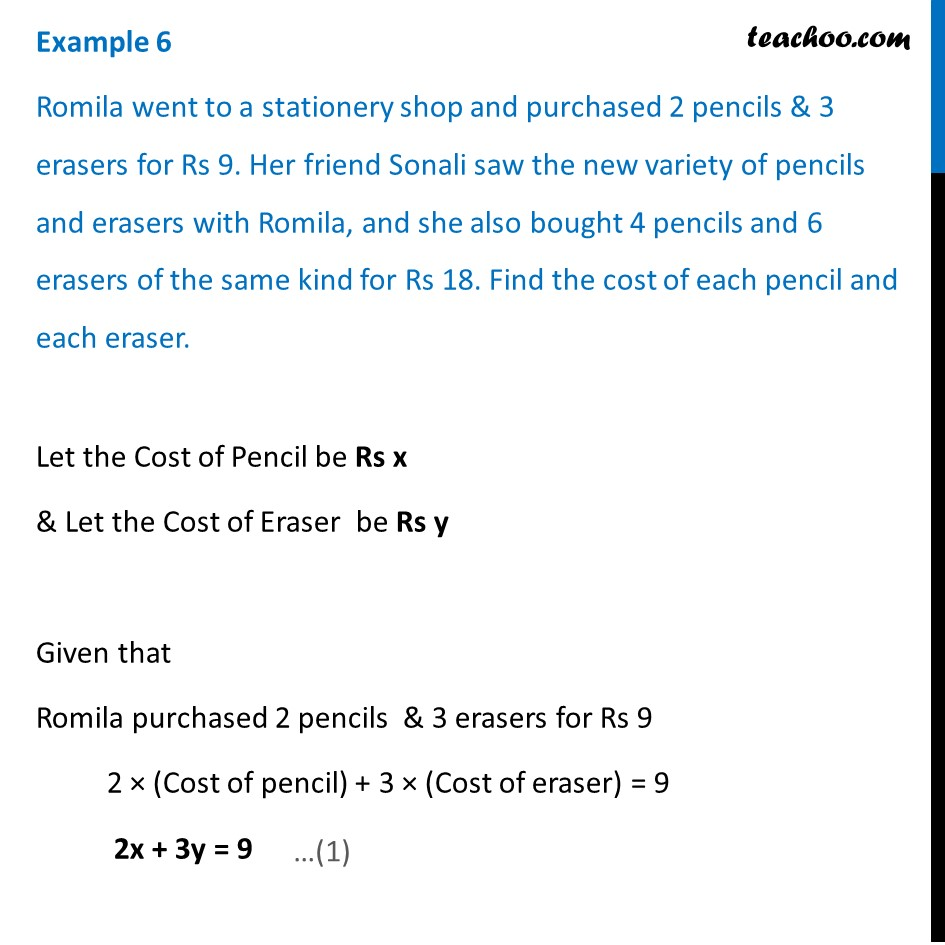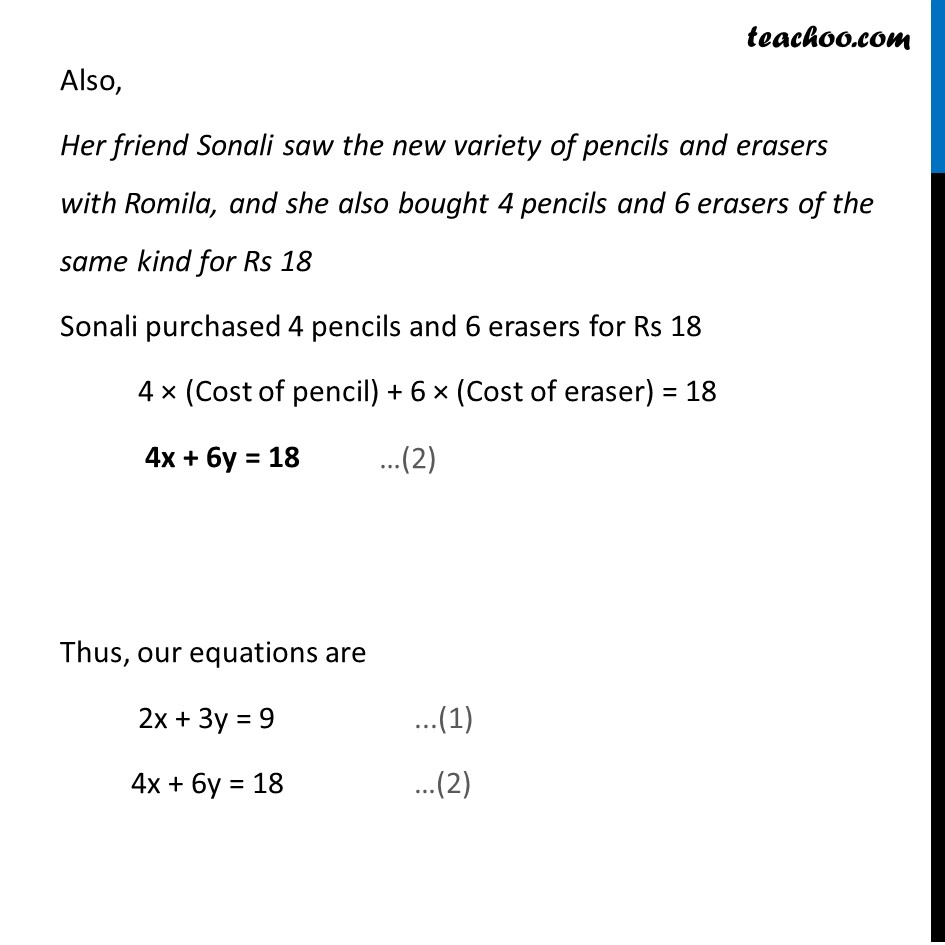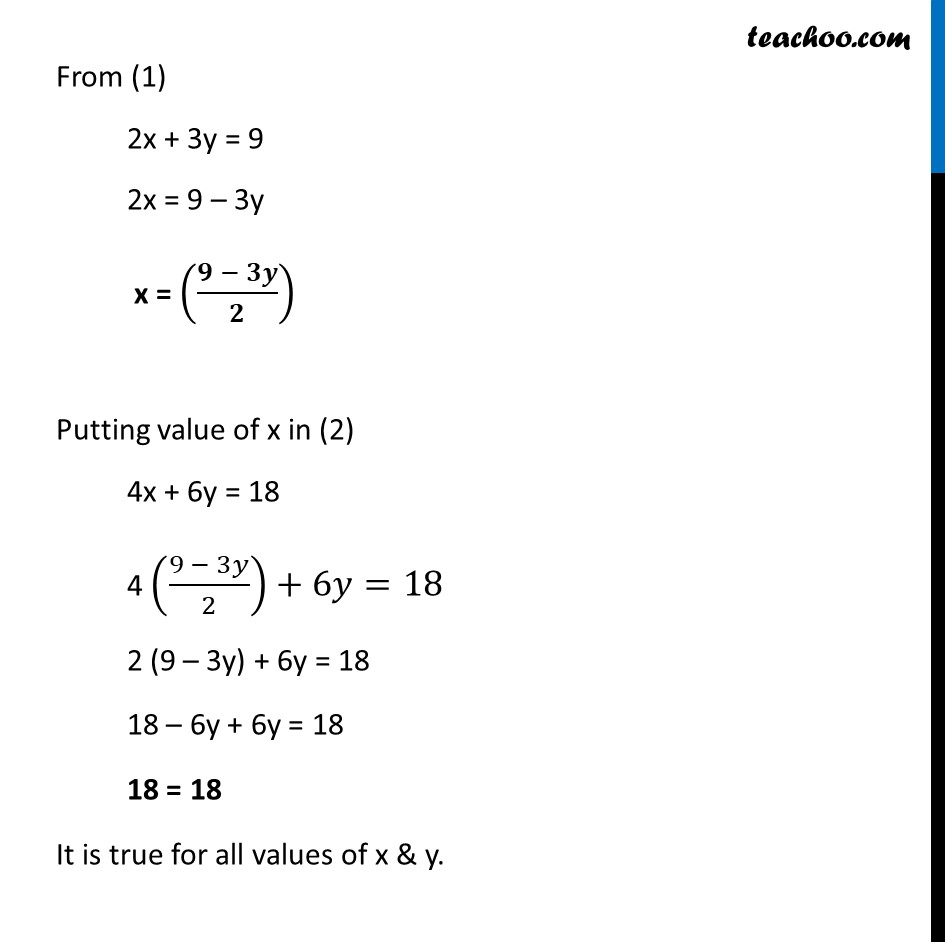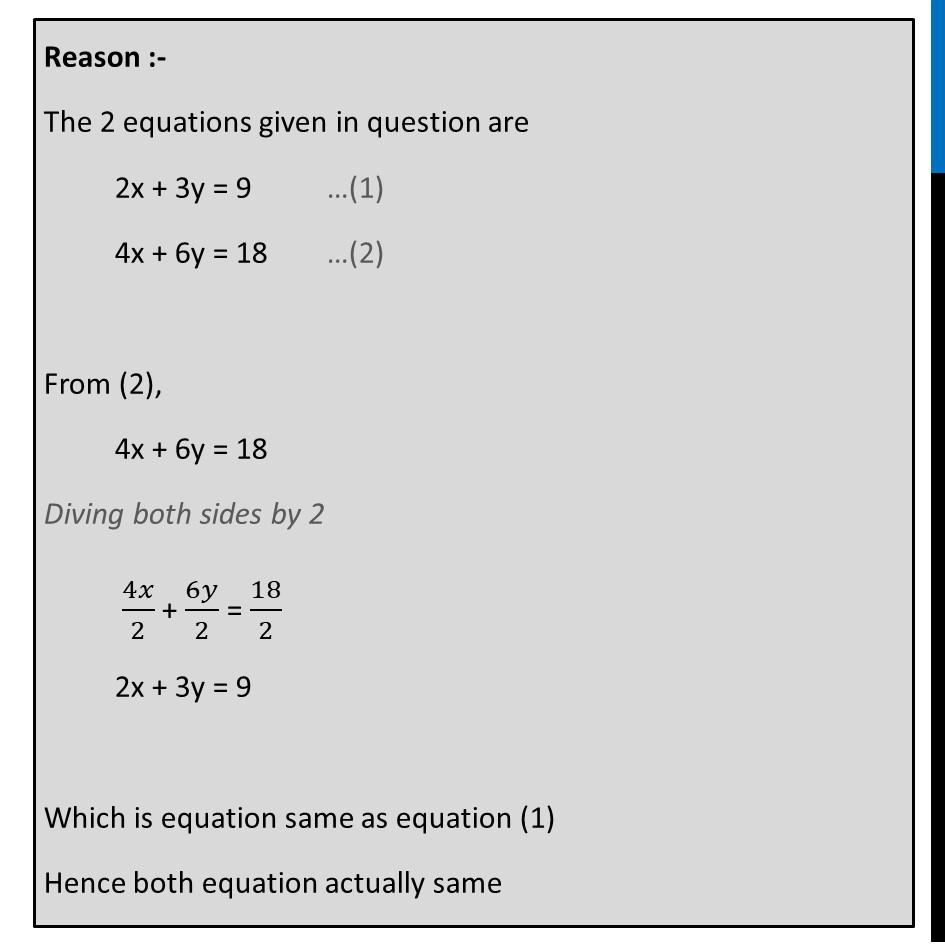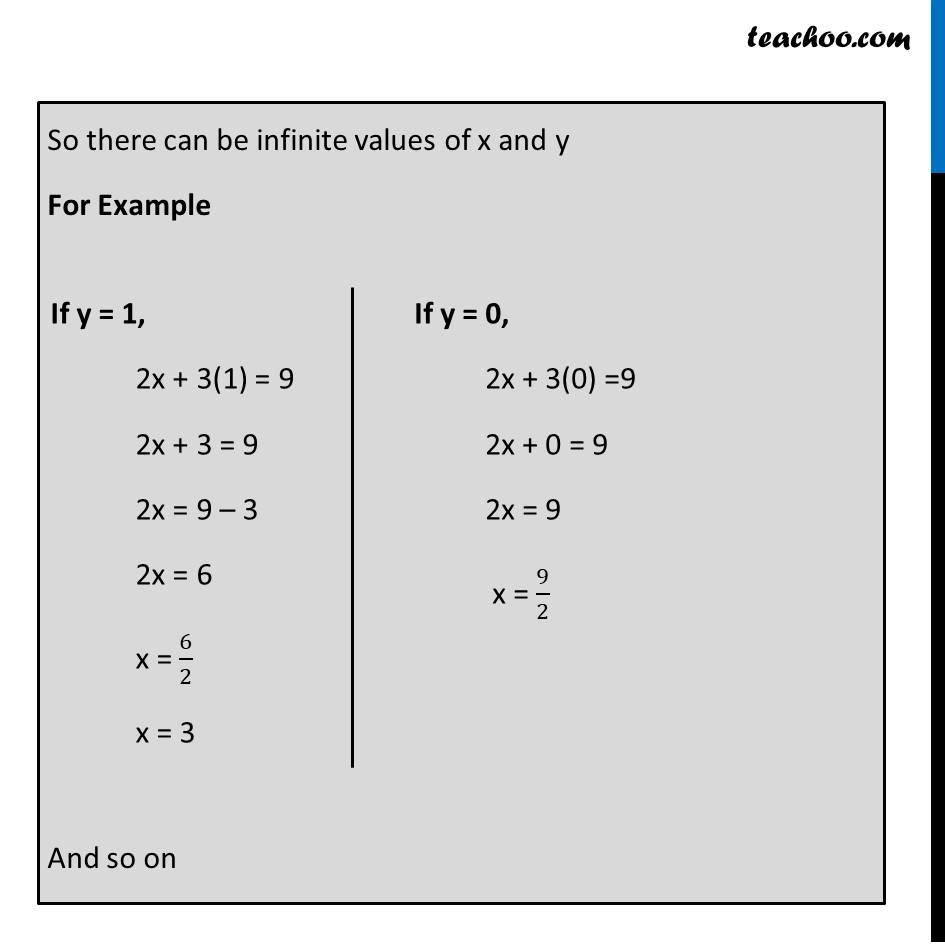Learn in your speed, with individual attention - Teachoo Maths 1-on-1 Class

### Transcript

Example 6 Romila went to a stationery shop and purchased 2 pencils & 3 erasers for Rs 9. Her friend Sonali saw the new variety of pencils and erasers with Romila, and she also bought 4 pencils and 6 erasers of the same kind for Rs 18. Find the cost of each pencil and each eraser. Let the Cost of Pencil be Rs x & Let the Cost of Eraser be Rs y Given that Romila purchased 2 pencils & 3 erasers for Rs 9 2 × (Cost of pencil) + 3 × (Cost of eraser) = 9 2x + 3y = 9 Also, Her friend Sonali saw the new variety of pencils and erasers with Romila, and she also bought 4 pencils and 6 erasers of the same kind for Rs 18 Sonali purchased 4 pencils and 6 erasers for Rs 18 4 × (Cost of pencil) + 6 × (Cost of eraser) = 18 4x + 6y = 18 Thus, our equations are 2x + 3y = 9 ...(1) 4x + 6y = 18 …(2) From (1) 2x + 3y = 9 2x = 9 – 3y x = ((𝟗 − 𝟑𝒚)/𝟐) Putting value of x in (2) 4x + 6y = 18 4 ((9 − 3𝑦)/2)+6𝑦=18 2 (9 – 3y) + 6y = 18 18 – 6y + 6y = 18 18 = 18 It is true for all values of x & y. Since the given equations have infinitely many solutions, That is why we cannot obtain a unique solution Since the given equations have infinitely many solutions, That is why we cannot obtain a unique solution